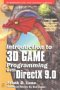# Maths - Vectors

 By: Nobody/Anonymous - nobodyInversion of line on plane projection   2005-07-16 02:07 Hi Martin. I'm trying to calculate the normal vector of a plane based on a known projection of a known 3D vector onto the plane.     Basically, I'm looking for the equation of a ray going from the closest point of a 3d cube (rendered to 2D projection) to my camera. I'm using the convention that this point is positioned at (0,0,0). Other visible points of the cube are at (1,0,0), (0,-1,0), (0,0,-1) and so forth. This would be simple were it possible to invert the cross product but i'm having a brain block. I hope this makes sense. Any ideas would be greatly appreciated. Thanks!    Jesse
 By: Martin Baker - martinbakerRE: Inversion of line on plane projection   2005-07-16 08:14 Hi Jesse,    There is no unique inverse of the vector cross product, this is not a deficiency with vector arithmetic, the same thing happens if we use equivalent matrices as shown here:  https://www.euclideanspace.com/maths/algebra/matrix/functions/skew/    This is accurately modeling the real world because if we cross multiply A x B to get a mutually perpendicular vector C, then we try to invert the problem to say that we know A and C and we want to find B we find that there are many values of B that will give us the answer we want so there is no solution.    If your problem does have a unique solution there must be a way to formulate it without using inverse cross product, or where the inverses cancel out?    Perhaps you could use projections of lines on planes or normals of lines to planes as shown here:  https://www.euclideanspace.com/maths/geometry/elements/plane/lineOnPlane/    Martin
 By: Nobody/Anonymous - nobodyRE: Inversion of line on plane projection   2006-01-15 00:51 Formulate the cube as it's dual, ie with planes.  You have the center vector C and the axis-normals to the cube faces A, such that the 8 vertices of the cube are    V[0..7] = C +/- A +/- A +/- A    Project these points onto the camera direction Z.    projection[0..7] = dot( V[0..7], Z ) = dot( C, Z ) +/- dot( A, Z ) +/- ... etc    The most extremal point along axis Z is obviously the one with all positive dot products, and the opposite the one with all negatives.    Thus, the closest point of cube C into the opposite direction of the camera is    C - A * abs( dot( A, Z ) ) - A * abs( dot( A, Z ) - A * abs( dot( A, Z ) )metadata block see also: Correspondence about this page Book Shop - Further reading. Where I can, I have put links to Amazon for books that are relevant to the subject, click on the appropriate country flag to get more details of the book or to buy it from them.Introduction to 3D Game Programming with DirectX 9.0 - This is quite a small book but it has good concise information with subjects like, maths introduction and picking.Mathematics for 3D game Programming - Includes introduction to Vectors, Matrices, Transforms and Trigonometry. (But no euler angles or quaternions). Also includes ray tracing and some linear & rotational physics also collision detection (but not collision response). Other Math Books Specific to this page here:

This site may have errors. Don't use for critical systems.